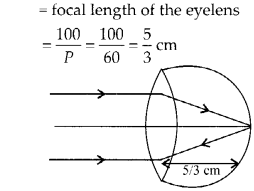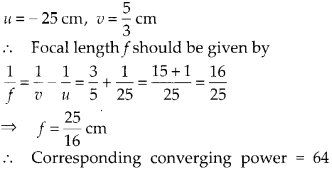Enlightened

# Question 24: NCERT Solutions for 12th Class Physics: Chapter 9-Ray Optics and Optical Instruments

• 0

Question 24: NCERT Solutions for 12th Class Physics: Chapter 9-Ray Optics and Optical Instruments

For a normal eye, the far points at infinity and the near point of distinct vision is about 25 cm in front of the eye. The cornea of the eye provides a converging power of about 40 dioptres, and the least converging power of the eye lens behind the cornea is about 20 dioptres. From this rough data estimate the range of accommodation (i.e., the range of converging power of the eye-lens) of a normal eye.

Share

1. Solution:
Here the least converging power of eye lens is given as 20 dioptres behind the cornea. If we can calculate the maximum converging power, then we can get the range of accommodation of a normal eye.
(a) To see objects at infinity, the eye uses its least converging power = 40 + 20 = 60 dioptres
∴ Approximate distance between the retina and the cornea eyelens(b) To focus object at the near point on the retina, we havedioptres Power of the eye lens = 64 – 40 = 24 dioptres Thus the range of accommodation of the eye lens is approximately 20 to 24 dioptres.Check the complete chapter with solutions.

NCERT Solutions for 12th Class Physics: Chapter 9-Ray Optics and Optical Instruments

• 0